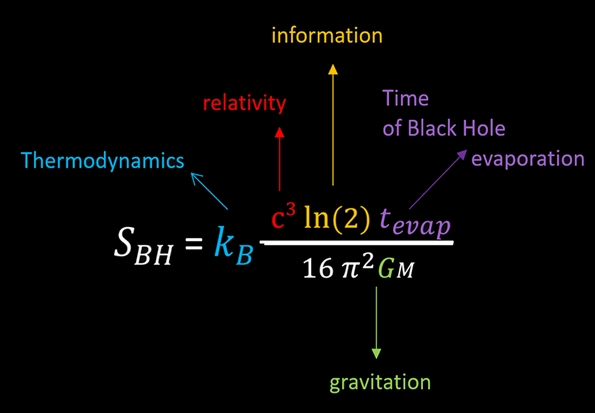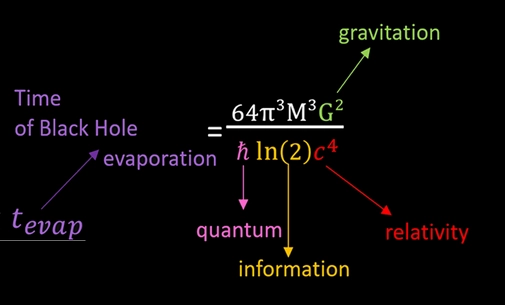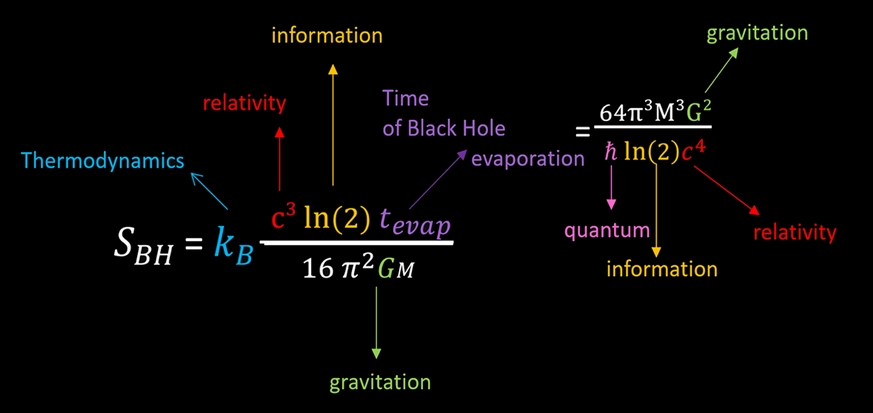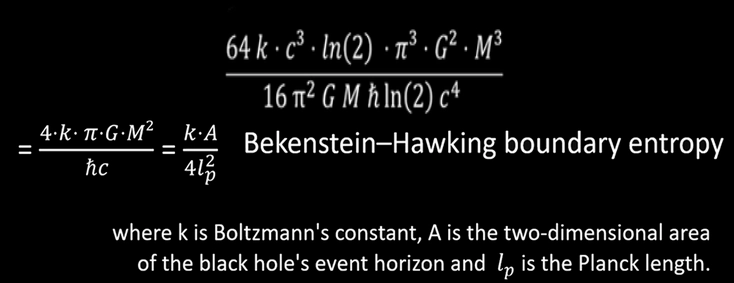# Black Hole entropic Information Formula

We start from the hidden thermodynamic of Louis De Broglie, where we replace the mass by the mass of bit of information.

$$ln(W)$$= $$action\over h$$=$$mc^2t \over h$$=$$kTln(2)t \over h$$

After we used this mass of bit of information at the Hawking temperature in the framework of the black hole thermodynamics and in the perspective of the Hawking radiation, the process of evaporation of the black hole.

With T = Hawking temperature

T= $$ħc^3\over 8πGkM$$

the Hawking Temperature, in relation to the storage of mass of bit of information;

$$m_{bit}$$=$$kTln(2)\over c^2$$

Black holes Entropic Information Formula with the time of evaporation of the black hole as new formulation of The Bekenstein–Hawking entropy additionally including the information notion;

so, the mass of bit of information is represented at Hawking Temperature in the frame of the Hawking radiation.

$$S_{BH}$$=$$(kc^3ln(2)t_{evap}) \over 16π^2GM$$The Hawking temperature formula which express itself by all the constant in modern physics bringing together: relativity, gravitation, quantum physics and thermodynamics.

With for the time of evaporation of the considered  black hole

$$t_{evap}$$=$$(64 π^3M^3G^2) \over ħln(2)c^4$$In the framework of Entropic Information, those formulas with the mass of bit of information must be taken in the frame of Black hole evaporating by Hawking radiation at the Hawking temperature with a time required to change the physical state of all the bit of information of the system equal to the time required by the black hole to evaporate.

To synthetize:We can see the black holes entropic information formula as new formulation of Bekenstein-Hawking entropy which is on the edge of thermodynamics, relativity, quantum, gravitation, but moreover, this new formulation takes in account the information theory

$$(64 π^3M^3G^2) \over (ħπ^2MGln(2)c^4)$$where k=Boltzmann constant, c=speed of light,  ħ = Reduced Planck's constant, G=Gravitational Constant, M= mass of the black hole and A is the two-dimensional area of the black hole’s event horizon, lp is the Planck length